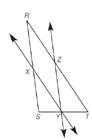Chapter 5.6, Problem 32EElementary Geometry For College St...

7th Edition
Alexander + 2 others
ISBN: 9781337614085

Solutions

Chapter
SectionElementary Geometry For College St...

7th Edition
Alexander + 2 others
ISBN: 9781337614085
Textbook Problem

Given: Δ R S T , with X Y ↔ ∥ R T ¯ and Y Z ↔ ∥ R S ¯Prove: R X X S = Z T R Z

To determine

To prove:

RXXS=ZTRZ

Explanation

Given:

In ΔRST, XYRT¯ and YZRS¯.

Property used:

If a line is parallel to one side of a triangle and intersects other two sides, then it divides these sides proportionally.

Proof:

Given: XYRT¯ and YZRS¯.

Consider XYRT¯

Still sussing out bartleby?

Check out a sample textbook solution.

See a sample solution

The Solution to Your Study Problems

Bartleby provides explanations to thousands of textbook problems written by our experts, many with advanced degrees!

Get Started

Find the mean, median, and mode for the following scores; 8, 7, 5, 7, 0, 10, 2, 4, 11, 7, 8, 7

Essentials of Statistics for The Behavioral Sciences (MindTap Course List)

Find y and y. y=1sect

Single Variable Calculus: Early Transcendentals, Volume I

Sketch the graph of y=x24.

Calculus: An Applied Approach (MindTap Course List)

The graph of is: a) b) c) d)

Study Guide for Stewart's Single Variable Calculus: Early Transcendentals, 8th